• +91 9971497814
• info@interviewmaterial.com

# RD Chapter 25- Probability Ex-25.1 Interview Questions Answers

### Related Subjects

Question 1 :
A coin is tossed 1000 times with the following frequencies:
Compute the probability for each event.

The coin is tossed 1000 times. So, the total number of trials is 1000.
Let A be the event of getting a head and B be the event of getting a tail.
The number of times A happens is 455 and the number of times B happens is 545.
Remember the empirical or experimental or observed frequency approach to probability.
If n be the total number of trials of an experiment and A is an event associated to it such that A happens in m-trials. Then the empirical probability of happening of event A is denoted by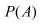and is given by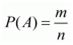Therefore, we have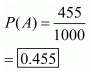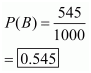Question 2 :
Two coins are tossed simultaneously 500 times with the following frequencies of different outcomes:
One tail: 290 times
Find the probability of occurrence of each of these events.

The total number of trials is 500.
Let A be the event of getting two heads, B be the event of getting one tail and C be the event of getting no head.
The number of times A happens is 95, the number of times B happens is 290 and the number of times C happens is 115.
Remember the empirical or experimental or observed frequency approach to probability.
If n be the total number of trials of an experiment and A is an event associated to it such that A happens in m-trials. Then the empirical probability of happening of event A is denoted byand is given byTherefore, we have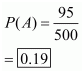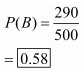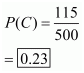Question 3 :
Three coins are tossed simultaneously 100 times with the following frequencies of different outcomes:

If the three coins are simultaneously tossed again, compute the probability of:
(iii) at least one head coming up.
(iv) getting more heads than tails.
(v) getting more tails than heads.

The total number of trials is 100.
Remember the empirical or experimental or observed frequency approach to probability.
If n be the total number of trials of an experiment and A is an event associated to it such that A happens in m-trials. Then the empirical probability of happening of event A is denoted byand is given by(i) Let A be the event of getting two heads.
The number of times A happens is 36.
Therefore, we have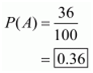(ii) Let B be the event of getting three heads
The number of times B happens is 12.
Therefore, we have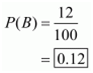(iii) Let C be the event of getting at least one head.
The number of times C happens is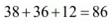.
Therefore, we have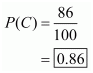(iv) Let D be the event of getting more heads than tails.
The number of times D happens is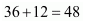.
Therefore, we have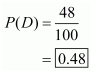(v) Let E be the event of getting more tails than heads.
The number of times E happens is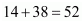.
Therefore, we have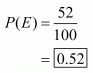Question 4 :
1500 families with 2 children were selected randomly and the following data were recorded:

 Number of girls in a family 0 1 2 Number of families 211 814 475
If a family is chosen at random, compute the probability that it has:
(i) No girl
(ii) 1 girl
(iii) 2 girls
(iv) at most one girl
(v) more girls than boys

The total number of trials is 1500.
Remember the empirical or experimental or observed frequency approach to probability.
If n be the total number of trials of an experiment and A is an event associated to it such that A happens in m-trials. Then the empirical probability of happening of event A is denoted byand is given by(i) Let A be the event of having no girl.
The number of times A happens is 211.
Therefore, we have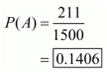(ii) Let B be the event of having one girl.
The number of times B happens is 814.
Therefore, we have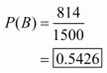(iii) Let C be the event of having two girls.
The number of times C happens is 475.
Therefore, we have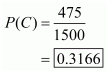(iv) Let D be the event of having at most one girl.
The number of times D happens is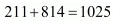.
Therefore, we have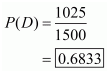(v) Let E be the event of having more girls than boys.
The number of times E happens is 475.
Therefore, we have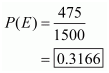Question 5 :
In a cricket match, a batsman hits a boundary 6 times out of 30 balls he plays.
(i) he hits boundary
(ii) he does not hit a boundary.

The total number of trials is 30.
Remember the empirical or experimental or observed frequency approach to probability.
If n be the total number of trials of an experiment and A is an event associated to it such that A happens in m-trials. Then the empirical probability of happening of event A is denoted byand is given by(i) Let A be the event of hitting boundary.
The number of times A happens is 6.
Therefore, we have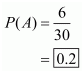(ii) Let B be the event of does not hitting boundary.
The number of times B happens is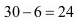.
Therefore, we have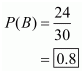Question 6 :
The percentage of marks obtained by a student in monthly unit tests are given below:

 Unit test: I II III IV V Percentage of marks obtained: 69 71 73 68 76
Find the probability that the student gets:
(i) more than 70% marks
(ii) less than 70% marks
(iii) a distinction

The total number of trials is 5.
Remember the empirical or experimental or observed frequency approach to probability.
If n be the total number of trials of an experiment and A is an event associated to it such that A happens in m-trials. Then the empirical probability of happening of event A is denoted byand is given by(i) Let A be the event of getting more than 70% marks.
The number of times A happens is 3.
Therefore, we have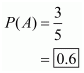(ii) Let B be the event of getting less than 70% marks.
The number of times B happens is 2.
Therefore, we have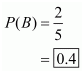(iii) Let C be the event of getting a distinction.
The number of times C happens is 1.
Therefore, we have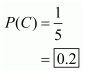Question 7 :
To know the opinion of the students about Mathematics, a survey of 200 students was conducted. The data is recorded in the following table:

 Opinion: Like Dislike Number of students: 135 65
Find the probability that a student chosen at random (i) likes Mathematics (ii) does not like it.

The total number of trials is 200.
Remember the empirical or experimental or observed frequency approach to probability.
If n be the total number of trials of an experiment and A is an event associated to it such that A happens in m-trials. Then the empirical probability of happening of event A is denoted byand is given by(i) Let A be the event of liking mathematics.
The number of times A happens is 135.
Therefore, we have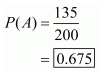(ii) Let B be the event of disliking mathematics.
The number of times B happens is 65.
Therefore, we have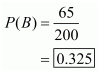Question 8 :
The blood groups of 30 students of class IX are recorded as follows:
 A B O O AB O A O B A O B A O O A AB O A A O O AB B A O B A B O

A student is selected at random from the class from blood donation, Fin the probability that the blood group of the student chosen is:
(i) A
(ii) B
(iii) AB
(iv) O

The total number of trials is 30.
Remember the empirical or experimental or observed frequency approach to probability.
If n be the total number of trials of an experiment and A is an event associated to it such that A happens in m-trials. Then the empirical probability of happening of event A is denoted byand is given by(i) Let A1 be the event that the blood group of a chosen student is A.
The number of times A1 happens is 9.
Therefore, we have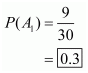(iii) Let A2 be the event that the blood group of a chosen student is B.
The number of times A2 happens is 6.
Therefore, we have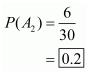(iii) Let A3 be the event that the blood group of a chosen student is AB.
The number of times A3 happens is 3.
Therefore, we have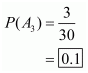(iv) Let A4 be the event that the blood group of a chosen student is O.
The number of times A4 happens is 12.
Therefore, we have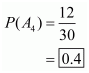Question 9 :
Eleven bags of wheat flour, each marked 5 Kg, actually contained the following weights of flour (in kg):
4.97, 5.05, 5.08, 5.03, 5.00, 5.06, 5.08, 4.98, 5.04, 5.07, 5.00

Find the probability that any of these bags chosen at random contains more than 5 kg of flour.

The total number of trials is 11.
Remember the empirical or experimental or observed frequency approach to probability.
If n be the total number of trials of an experiment and A is an event associated to it such that A happens in m-trials. Then the empirical probability of happening of event A is denoted byand is given byLet A1 be the event that the actual weight of a chosen bag contain more than 5 Kg of flour.
The number of times A1 happens is 7.
Therefore, we have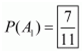Question 10 :
Following table shows the birth month of 40 students of class IX.

 Jan. Feb March April May June July Aug. Sept. Oct. Nov. Dec. 3 4 2 2 5 1 2 5 3 4 4 4
Find the probability that a student was born in August.

The total number of trials is 40.
Remember the empirical or experimental or observed frequency approach to probability.
If n be the total number of trials of an experiment and A is an event associated to it such that A happens in m-trials. Then the empirical probability of happening of event A is denoted byand is given byLet A1 be the event that the birth month of a chosen student is august.
The number of times A1 happens is 5.
Therefore, we have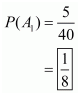Todays Deals### RD Chapter 25- Probability Ex-25.1 Contributorskrishan

Name:
Email:

# Latest News# 9000 interview questions in different categories